# Perform IF not this or that using formula in Microsoft Excel

In this article, we will learn how to perform IF function with specifically segregate using NOT function

In simple words, In a list of values perform a function where not required values need not be considered.

IF function in Excel is used to check the condition and returns value on the basis of it.
Syntax:

=IF(Logical_test, [Value_if True], [Value_if_False])

The NOT function returns the opposite boolean value. NOT function in excel returns TRUE if False and False if TRUE.
Syntax:

=NOT(Logical)

Logical: Boolean value or Logic_test
OR function works on logic_test. It helps you run multiple conditions in Excel. If anyone of them is True, OR function returns True else False.
Syntax:

=OR( Logical 1, [Logical 2], ..)

Let’s understand this using the above functions.’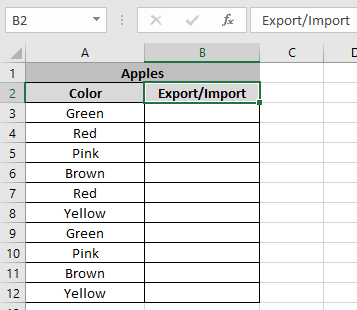Here we need to segregate data on the basis of colour of apples to import or export.

We don’t want Green & Brown colour to export.

So we will be using a formula:

=IF (NOT (OR (A3="Green", A3="Brown")), "Export", "Import")

Explanation:
Here OR function checks the cell for text “Green” or “Brown” Apples.
NOT function returns False for Green or Brown Apples and True elsewhere.
“Import” : Value_if_true
“Export” : value_if_false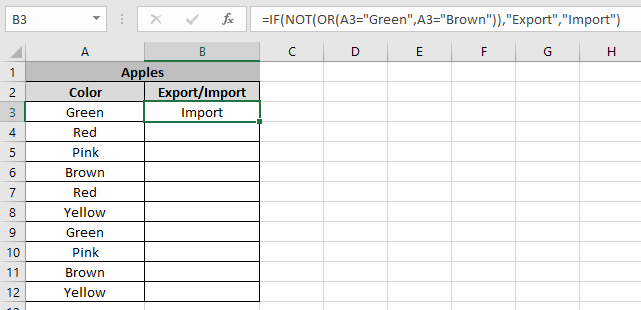The formula returns Import. That’s correct

Now copy the formula to other cells using shortcut Ctrl + D.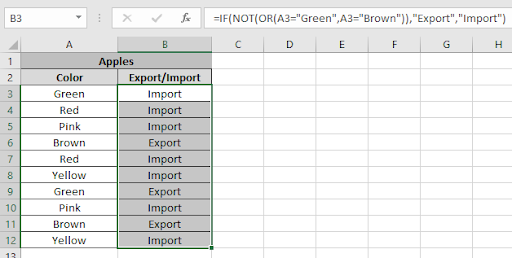As you can see the formula works fine. Combination of IF , NOT & OR function segregate Apples where different operations need to be performed.

Now we need to perform tasks using the above method.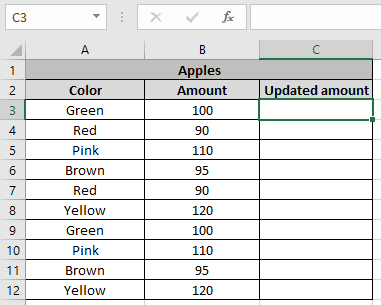Here we need to update the amount for different types of Apples.

Green & Brown colour apples amount needs to increase by 10% and remain same elsewhere.

Use the formula for this:

=IF (NOT (OR (A3="Green", A3="Brown")), B3, B3*1.1)

Here OR function checks the cell for text “Green” or “Brown” Apples.
NOT function returns False for Green or Brown Apples and True elsewhere.
B3 : Value remain same if true
B3*1.1 : value increased by 10% if false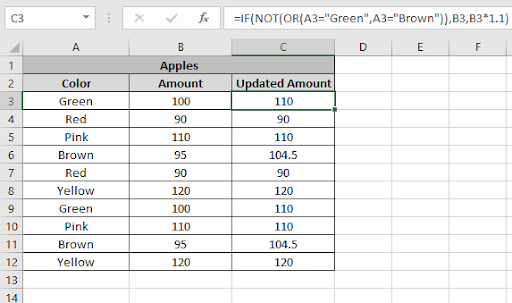As you can see we updated the amount using the Logic function in excel.

Hope you understood IF, NOT and OR function in Excel 2016. Find more articles on Excel Logic_test functions here. Please share your query below in the comment box. We will assist you.

Related Articles:

IF with AND Function in Excel

IF with AND and OR function in Excel

Excel Operations If Not Equal To in Excel

Count Cells That Contain This Or That in Excel

Popular Articles:

How to use the VLOOKUP Function in Excel

How to use the COUNTIF function in Excel

How to use the SUMIF Function in Excel

Terms and Conditions of use

The applications/code on this site are distributed as is and without warranties or liability. In no event shall the owner of the copyrights, or the authors of the applications/code be liable for any loss of profit, any problems or any damage resulting from the use or evaluation of the applications/code.